On this page, you will find Electricity Class 10 Notes Science Chapter 12 Pdf free download. CBSE NCERT Class 10 Science Notes Chapter 12 Electricity will seemingly help them to revise the important concepts in less time.

## CBSE Class 10 Science Chapter 12 Notes Electricity

### Electricity Class 10 Notes Understanding the Lesson

1. Current electricity: The branch of physics which deals with the study of charge in motion is called current electricity.

2. Electric charge: Charge is the property of matter due to which it produces and experiences electric and magnetic effects.

• SI unit of charge is coulomb (C).
• There exists two types of charges in nature.

(i) positive charge
(ii) negative charge

• Smallest stable charged particles are protons and electrons.
• Charge on an electron is negative and that on a proton is positive but both are equal in magnitude
i. e., 1.6 x 10-19C

Properties of charge

• Like charges repel each other and unlike charges attract each other.
• Charge can neither be created nor destroyed but it can be transferred from one body to another
i. e., charge is conserved.

3. Electric current (I): Electric current is defined as the total amount of charge flowing through a particular area in unit time. It is denoted by T. $$I=\frac{Q}{t}$$

where, Q = amount of charge, t = time interval

• Electric current is a scalar quantity.
• SI unit of current is ampere (A).
• 1 Ampere

One ampere is constituted by the flow of one coulomb of charge per second.
$$I=\frac{Q}{t} \mid \text { If } Q=1 C \text { and } t=1 s, \text { then } I=\frac{1 C}{1 S}=1 A$$

• Small quantities of current are expressed in milliampere or in microampere.
1 mA = 10-3 A (Here ‘m’ is a prefix used to express milli)
10 μA = 10-6 A (‘μ’ is symbol of micro)

4. Direction of current:

• Electric current flows in the circuit from positive terminal of cell to the negative terminal of cell.
• In metals flow of electron constitutes current. Conventionally the direction of current is taken as opposite to the direction of motion of electrons.

5. Electric potential difference: Electric potential difference between two points in an electric circuit, carrying current can be defined as the amount of work done to move a unit charge from one point to another.
$$\text { Potential difference }(\mathrm{V})=\frac{\text { Work done }(\mathrm{W})}{\text { Charge }(\mathrm{Q})}$$
$$V=\frac{W}{Q}$$

• SI unit of potential difference is volt (V).
• The electron moves in a wire due to potential difference. The potential difference may be produced by chemical reaction occurring in a battery.
• 1 volt (1 V)

One volt is the potential difference between two points in a current carrying conductor, when 1 Joule of work is done to move a charge of 1 coulomb from one point to the other.
$$\text { Therefore, } 1 \text { volt }=\frac{1 \text { Joule }}{1 \text { Coulomb }}$$
IV = 1JC-1

6. Electric circuit: A continuous and closed path of electric current is called an electric circuit.

7. Circuit diagram: A circuit is a simplified systematic representation of the components of an electrical circuit.
Table 12.1 Symbols of some commonly used components in circuit diagrams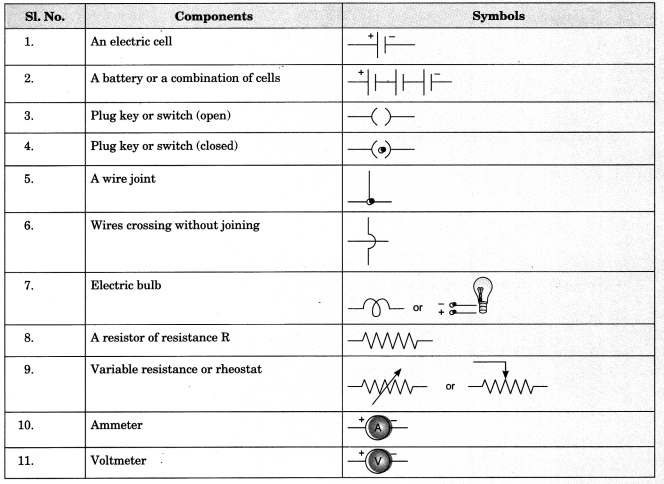8. Measuring Instruments:

• Ammeter: It is used to measure the magnitude of electric current through any wire of a circuit.
• It is always connected in series.
• It should have low resistance.

Voltmeter: It measures the potential difference between two points of the circuit.

• The voltmeter is always connected in parallel across the points between which the potential difference to be measured.
• It should have high resistance.

9. Ohm’s law: According to ohm’s law, the potential difference, v, across the ends of a given metallic wire in an electric circuit is directly proportional to the current flowing.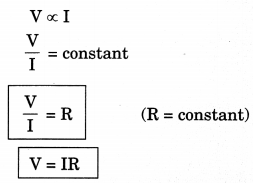Here, R is constant for a given metallic wire at a given temperature and is called resistance.

10. V-I graph of ohm’s law and its experimental setup:
1. Circuit diagram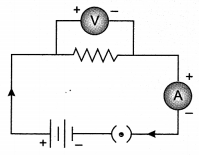2. Variation of current with potential difference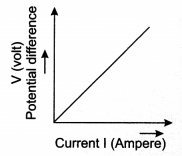Slope of V-I curve gives resistance
$$R=\text { slope }=\frac{\Delta \mathrm{V}}{\Delta \mathrm{I}}$$
I-V curve of Ohm’s law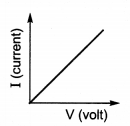Slope of I-V curve =$$\frac{1}{\mathrm{R}}$$
$$\frac{1}{\mathrm{R}}=\text { Slope }=\frac{\Delta \mathrm{I}}{\Delta \mathrm{V}}$$

11. Resistance:

• It is the property of a conductor to resist the flow of charges through it.
• SI unit of resistance is ohm, represented by Greek letter Ω (omega).

(i) One ohm
If the potential difference across the two ends of a conductor is IV and the current through it is 1A, then the resistance, R, of the conductor is 1 Ω.
$$1 \mathrm{ohm}=\frac{1 \text { volt }}{1 \mathrm{ampere}}$$

• The current through a resistor is inversely proportional to its resistance keeping voltage constant.
• Resistance in a circuit arises due to retardation in motion of electrons as they are restrained by attraction of atom among which they move.

(ii) Rheostat
Rheostat is a component or device used to regulate current without changing the voltage source, it is also called variable resistance.

12. Factors affecting resistance of a conductor

• Resistance of a uniform metallic conductor is directly proportional to its length (l)
R α Z …………(1)
• Resistance is inversely proportional to area of cross-section (A).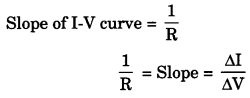where, ρ(rho) is constant of proportionality and it is called electric resistivity of the material of the conductor.

• Resistance of a metallic conductor also depends on temperature.
• It also depends on the nature of the material.

13. Electric resistivity (ρ)
(i) Resistivity depends on the nature of the material and temperature. It is independent of the length and area of cross-section of the conductor.

(ii) where, R = resistance
A = Area of cross-section
l = length of conductor

(iii) SI unit of resistivity is ‘Ωm’.

14. Classification of Elements on the basis of resistivity

• Metals and alloys have very low resistivity in the range of 10-8 O m to 10-6 Q m. They are good conductors of electricity.
• Insulators have resistivity of the order of 1012 to 1017 Q m. They are bad conductors of electricity.

15. Use of elements on the basis of resistivity.

• Copper and aluminium are used for electric transmission lines because both posses low resistivity.
• Alloys are commonly used in electric heating appliances.

Resistivity of an alloy is generally higher than that of its constituent metals. Alloy do not oxidise readily at high temperatures. For this reason, they are commonly used in electric heating devices.

16. Combination of Resistors17. Special Case

 Resistance in Series Resistance in Parallel When ‘n’ number of identical resistors having resistance ‘R’ is connected in series then equivalent resistance becomes $$R_{e q}=n \mathrm{R}$$ When ‘n’ number of identical resistors having resistance ‘R’ is connected in parallel then equivalent resistance becomes$$\mathrm{R}_{e q}=\frac{\mathrm{R}}{n}$$

18. Some Important points for series and parallel combination

• For getting maximum equivalent resistance, all resistors should be connected in series and for getting minimum equivalent resistance, all resistors should be connected in parallel.
• In domestic circuits, parallel combination is used because in series arrangement, if any one of appliances fails or is switched off, all the other appliances stop working.

19. Electric power (P): The rate at which electric energy is consumed or dissipated is called electric power.
Electric power
$$P=V I=I^{2} R=\frac{V^{2}}{R}$$

• One watt (1 W)
One watt is the power consumed by a device that carries 1 A of current when operated at a potential difference of 1 V.
Thus, 1 W = 1 volt x 1 ampere = 1 V A
• Larger unit of power
1 kW = 1000 W (1 kW = one kilowatt)
• Commercial unit of electric energy (kWh) (units)
1 KWh = 1000 watt x 3600 seconds
1 unit = 1 kWh = 3.6 x 106 J

One kilowatt hour is the energy consumed when 1 kilowatt of power is used for one hour.

Heating effect of electric current

• Heating effect: When an electric current passes through a wire, the wire gets heated and its temperature rises. This is known as heating effect of electric current.

Joule’s law of heating: Heat produced in a conductor is directly proportional to

• square of current for a given resistor (H α I2)
• the resistance for a given current (H α R)
• the time for which the current flows through the resistor (H α t) H = I2Rf

20. Practical applications of heating effect of electric current

1. Incandescent electric lamp: The heating effect of electric current is also used to produce light, like in a electric bulb. The filament of the bulb is made up of tungsten with high melting point (3380°C). It is thermally isolated using insulated support. Presence of chemically inactive gases like argon and nitrogen prolong the life of the filament. When voltage is applied across the filament of the bulb, the current starts passing through it. The filament gets heated to a very high temperature (2700°C). It becomes white hot and starts radiating heat and light.

2. Fuse: Fuse is a device which is based on the principal of heating effect of electric current. It protects circuits and appliances by stopping the flow of any unduly high current. It consists a piece of wire made of a metal or an alloy of appropriate melting point. If a current larger than the specified value flows through the circuit, the temperature of the fuse wire increases. This melts the fuse wire and breaks the circuit.

### Class 10 Science Chapter 12 Notes Important Terms

Current (I): The rate of flow of charge is called current.

Potential Difference (V): Work done to move a unit charge from one point to another.

Volt: When 1 joule work is done in carrying one coulomb charge then potential difference is called 1 volt. Voltmeter: Instrument used to measure potential difference.

Ammeter: Instrument used to measure electric current.

Ohm’s Law: Potential difference across two points of a metallic conductor is directly proportional to current passing through the circuit provided that temperature remains constant.

Resistance (R): It is the property of a conductor to resist the flow of charges through it.

Rheostat: Variable resistance is a component used to regulate current without changing the source of voltage.

Resistivity (ρ): It is defined as the resistance offered by a cube of a material of side 1 m when current flows perpendicular to its opposite faces.

Electric Fuse: It is a safety device that protects our electrical appliances in case of short circuit or overloading

Electric Power: The rate at which electric energy is consumed or dissipated in an electric circuit.

Heating Effect of Electric Circuit: If an electric circuit is purely resistive, the source of energy continually gets dissipated entirely in form of heat. This is known as heating effect of electric current.